##### Trigonometry Workbook For DummiesYou can use the sum and difference formulas for cosine to calculate the cosine of the sums and differences of angles similarly to the way you can use the sum and difference formulas for sine, because the formulas look very similar to each other. When working with sines and cosines of sums and differences of angles, you're simply plugging in given values for the variables (angles). Just make sure you use the correct formula based on the information you're given in the question.

Here are the sum and difference formulas for cosines: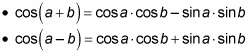The sum and difference formulas for cosine (and sine) can do more than calculate a trig value for an angle not marked on the unit circle (at least for angles that are multiples of 15 degrees). They can also be used to find the cosine (and sine) of the sum or difference of two angles based on information given about the two angles. For such problems, you'll be given two angles (call them A and B), the sine or cosine of A and B, and the quadrant(s) in which the two angles are located.

Use the following steps to find the exact value of cos(A + B), given that cos A = –3/5, with A in quadrant II of the coordinate plane, and sin B = –7/25, with B in quadrant III:

1. Choose the appropriate formula and substitute the information you know to determine the missing information.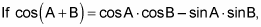then substitutions result in this equation: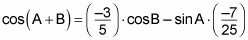To proceed any further, you need to find cos B and sin A.

2. Draw pictures representing right triangles in the quadrant(s).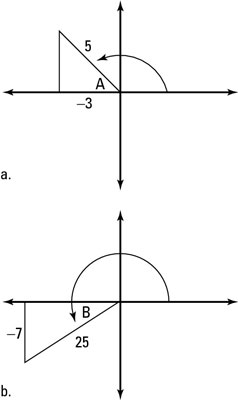Drawing pictures helps you visualize the missing pieces of info.

You need to draw one triangle for angle A in quadrant II and one for angle B in quadrant III. Using the definition of sine as opp/hyp and cosine as adj/hyp, this figure shows these triangles. Notice that the value of a leg is missing in each triangle.

3. To find the missing values, use the Pythagorean theorem.

The length of the missing leg in Figure a is 4, and the length of the missing leg in Figure b is –24.

4. Determine the missing trig ratios to use in the sum or difference formula.

You use the definition of cosine to find that cos B = –24/25 and the definition of sine to find that sin A = 4/5.

5. Substitute the missing trig ratios into the sum or difference formula and simplify.

You now have this equation: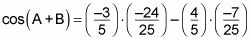Follow the order of operations to get this answer: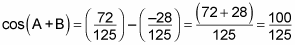This equation simplifies to cos(A + B) = 4/5.